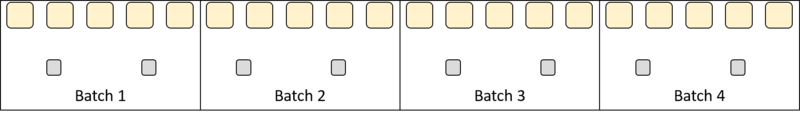##### Engage your students with effective distance learning resources. ACCESS RESOURCES>>

Alignments to Content Standards: 6.RP.A

A bread recipe calls for 5 cups of flour and 2 teaspoons of yeast.

1. How much flour and how much yeast do you need for two batches of bread? Draw a picture that shows the batches as well as the total amount of flour and total amount of yeast needed.
2. How many batches can you make with 15 cups of flour and 6 teaspoons of yeast? Indicate the batches in the picture.3. We say that the ratios 5:2, 10:4, and 15:6 are equivalent. In this case, even though the amounts of each ingredient are not the same, they would make the same kind of bread. Find another ratio equivalent to these (and that would correspond to the same kind of bread) and draw a picture to represent it.

## IM Commentary

The purpose of this task is to provide students with a first informal experience with the idea of equivalent ratios. The intuitive notion that making multiple batches of the same recipe results in the same bread as a single recipe helps motivate the idea that different ratios can be "the same" in some important way. Later, students will formalize the idea that a : b is equivalent to any ratio sa : sb, where s > 0.

The task statement shows different-sized blobs, so that cups are represented with larger blobs and teaspoons are represented with smaller blobs, although the blob sizes are not proportional in any way to actual cups and teaspoons.  Note that a student might produce a correct drawing where all the blobs are the same size or the relative sizes of the units are not reflected in the diagram. The important thing is that the correct values are shown for each quantity in the ratio.

A possible followup task could be:

Another recipe calls for 2 cups of flour and 1 teaspoon of yeast.

1. How much flour and how much yeast do you need for two batches of this bread?
2. Compare two batches of this bread with one batch of the first bread recipe. Do you think these ratios are equivalent?

Students might say that since they make different kinds of bread since the amount of flour is different when the amount of yeast is the same, the ratios are not equivalent.

## Solution

1. You need 10 cups of flour and 4 tablespoons of yeast for two batches of bread because you double all of the ingredients.2. You can make 3 batches, as indicated in the picture.3. Another equivalent ratio is 20:8, which is what you get with four batches of bread.